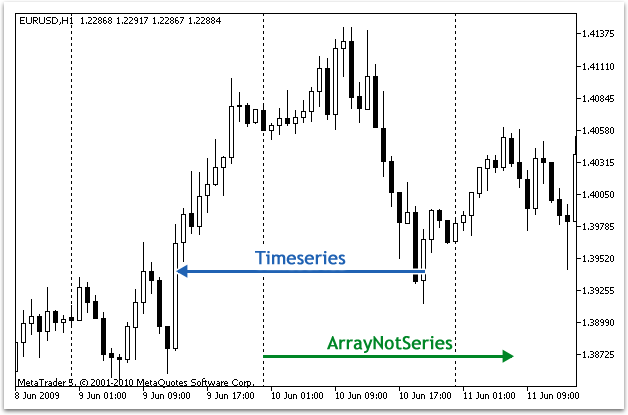MQL5参考时间序列和指标访问

# 访问时间序列和指标数据SeriesInfoInteger

Bars

BarsCalculated

IndicatorCreate

IndicatorParameters

IndicatorRelease

CopyBuffer

CopyRates

CopySeries

MqlRates结构获取指定交易品种周期和指定数量的同步时间序列。数据被收入到指定的数组集中。元素从现在到过去倒数，这意味着起始位置等于0表示当前柱形图。

CopyTime

CopyOpen

CopyHigh

CopyLow

CopyClose

CopyTickVolume

CopyRealVolume

CopyTicks

CopyTicksRange

iBars

iBarShift

iClose

iHigh

iHighest

iLow

iLowest

iOpen

iTime

iTickVolume

iRealVolume

iVolume

 datetime TimeAsSeries[]; //--- 如时间序列访问一样设置数组访问    ArraySetAsSeries(TimeAsSeries,true);    ResetLastError();    int copied=CopyTime(NULL,0,0,10,TimeAsSeries);    if(copied<=0)      {       Print("The copy operation of the open time values for last 10 bars has failed");       return;      }    Print("TimeCurrent =",TimeCurrent());    Print("ArraySize(Time) =",ArraySize(TimeAsSeries));    int size=ArraySize(TimeAsSeries);    for(int i=0;i=0;i--)      {       Print("ArrayNotSeries["+i+"] =",ArrayNotSeries[i]);      }

 TimeCurrent = 2009.06.11 14:16:23 ArraySize(Time) = 10 TimeAsSeries = 2009.06.11 14:00:00 TimeAsSeries = 2009.06.11 13:00:00 TimeAsSeries = 2009.06.11 12:00:00 TimeAsSeries = 2009.06.11 11:00:00 TimeAsSeries = 2009.06.11 10:00:00 TimeAsSeries = 2009.06.11 09:00:00 TimeAsSeries = 2009.06.11 08:00:00 TimeAsSeries = 2009.06.11 07:00:00 TimeAsSeries = 2009.06.11 06:00:00 TimeAsSeries = 2009.06.11 05:00:00   ArrayNotSeries = 2009.06.11 14:00:00 ArrayNotSeries = 2009.06.11 13:00:00 ArrayNotSeries = 2009.06.11 12:00:00 ArrayNotSeries = 2009.06.11 11:00:00 ArrayNotSeries = 2009.06.11 10:00:00 ArrayNotSeries = 2009.06.11 09:00:00 ArrayNotSeries = 2009.06.11 08:00:00 ArrayNotSeries = 2009.06.11 07:00:00 ArrayNotSeries = 2009.06.11 06:00:00 ArrayNotSeries = 2009.06.11 05:00:00